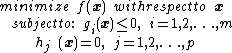# nonlinear programming

(redirected from Nonlinear optimization)

## nonlinear programming

[′nän‚lin·ē·ər ′prō‚gram·iŋ]
(mathematics)
A branch of applied mathematics concerned with finding the maximum or minimum of a function of several variables, when the variables are constrained to yield values of other functions lying in a certain range, and either the function to be maximized or minimized, or at least one of the functions whose value is constrained, is nonlinear.
McGraw-Hill Dictionary of Scientific & Technical Terms, 6E, Copyright © 2003 by The McGraw-Hill Companies, Inc.

## Nonlinear programming

The area of applied mathematics and operations research concerned with finding the largest or smallest value of a function subject to constraints or restrictions on the variables of the function. Nonlinear programming is sometimes referred to as nonlinear optimization.

A useful example concerns a power plant that uses the water from a reservoir to cool the plant. The heated water is then piped into a lake. For efficiency, the plant should be run at the highest possible temperature consistent with safety considerations, but there are also limits on the amount of water that can be pumped through the plant, and there are ecological constraints on how much the lake temperature can be raised. The optimization problem is to maximize the temperature of the plant subject to the safety constraints, the limit on the rate at which water can be pumped into the plant, and the bound on the increase in lake temperature.

The nonlinear programming problem refers specifically to the situation in which the function to be minimized or maximized, called the objective function, and the functions that describe the constraints are nonlinear functions. Typically, the variables are continuous; this article is restricted to this case.

Researchers in nonlinear programming consider both the theoretical and practical aspects of these problems. Theoretical issues include the study of algebraic and geometric conditions that characterize a solution, as well as general notions of convexity that determine the existence and uniqueness of solutions. Among the practical questions that are addressed are the mathematical formulation of a specific problem and the development and analysis of algorithms for finding the solution of such problems.

The general nonlinear programming problem can be stated as that of minimizing a scalar-valued objective function f(x) over all vectors x satisfying a set of constraints. The constraints are in the form of general nonlinear equations and inequalities. Mathematically, the nonlinear programming problem may be expressed as below,where x = (x1, x2, …, xn) are the variables of the problem, f is the objective function, gi( x ) are the inequality constraints, and hj( x ) are the equality constraints. This formulation is general in that the problem of maximizing f( x ) is equivalent to minimizing -f( x ) and a constraint gi( x ) ≥ 0 is equivalent to the constraint -gi( x ) ≤ 0.

Since general nonlinear equations cannot be solved in closed form, iterative methods must be used. Such methods generate a sequence of approximations, or iterates, that will converge to a solution under specified conditions. Newton's method is one of the best-known methods and is the basis for many of the fastest methods for solving the nonlinear programming problem.

McGraw-Hill Concise Encyclopedia of Engineering. © 2002 by The McGraw-Hill Companies, Inc.
References in periodicals archive ?
Next the call of the built-in function of unconditional nonlinear optimization fminsearch() according to the deformable polyhedron method  comes.
To reduce the computation time and resources for the nonlinear optimization on full vehicle model with a large number of design variables, the equivalent static loads (ESL) method was used to run the structural optimization analysis.
Single Valued Neutrosophic Hesitant Fuzzy Computational Algorithm for Multiobjective Nonlinear Optimization Problem.
Since the calculated moments for the gPC expansion are nonlinear functions of the coefficients, one has to employ nonlinear optimization procedure.
The enhanced version addresses one of the problems of CSO, that is, the fact that it is often trapped in local optima in nonlinear optimization problems with a large number of local extreme values.
When SVM is used in the two classification problems, the original model can be written as the following nonlinear optimization problem:
Different types of open-source and licensed optimizers that solve the general nonlinear optimization problem have been integrated into the package, such as SNOPT, SLSQP, and ALPSO.
A computational algorithm, based on nonlinear optimization techniques and numerical methods for solving boundaryvalue ordinary differential equations, is then proposed.
These optimization problems include game theory, linear programming problems, linear complement problems, projection equation problems, variational inequality problems, nonlinear optimization problems, general convex optimization problems, nonconvex optimization problems, and nonsmooth optimization problems.
However, the key point of nonlinear optimization is not to obtain the exact flutter speed but to evaluate the dynamic stability at the flutter constraint.
Toint, "Recent progress in unconstrained nonlinear optimization without derivatives," Mathematical Programming, vol.

Site: Follow: Share:
Open / Close